# Decision tree and RapidMiner performance measures - how to understand them

Member Posts: 11Contributor II
I would like to ask for help in the following matter.
In a decision tree created with gain ratio I just receive the classification of every instance to some class. In my case, one of 2 classes.
I do not understand how the RMSE is calculated if this measure is based on the difference between actual value and predicted value. If my classes use index symbols 0 and 1, does it mean that always the difference is 0 or 1 between actual value and predicted value?
Similarly, I do not undestand the margin definition. The margin is defined as the minimal confidence for the correct label. Should I calculate confidence for all the nodes and take the minimum value?
Finally, I do not understand the soft margin.Soft margin loss is the average soft margin loss on a classifier defined as the average of all 1- confidences for the correct label. How do I caculate 1-confidence for the correct label?

Tagged:

• Administrator, Employee, RapidMiner Certified Analyst, RapidMiner Certified Expert, Member Posts: 363RM Data Scientist
edited February 2020
Hi @Picia,

If  you have a binary label (0 or 1) for prediction with Decision Tree, the best way is to convert the target type from numeric to nominal and apply "performance (Binomial Classification)" operator to extract the measurements for classification models.
AUC, classification error, accuracy, recall, F-measurement, ect. are usually the metrics used for Binomial Classification.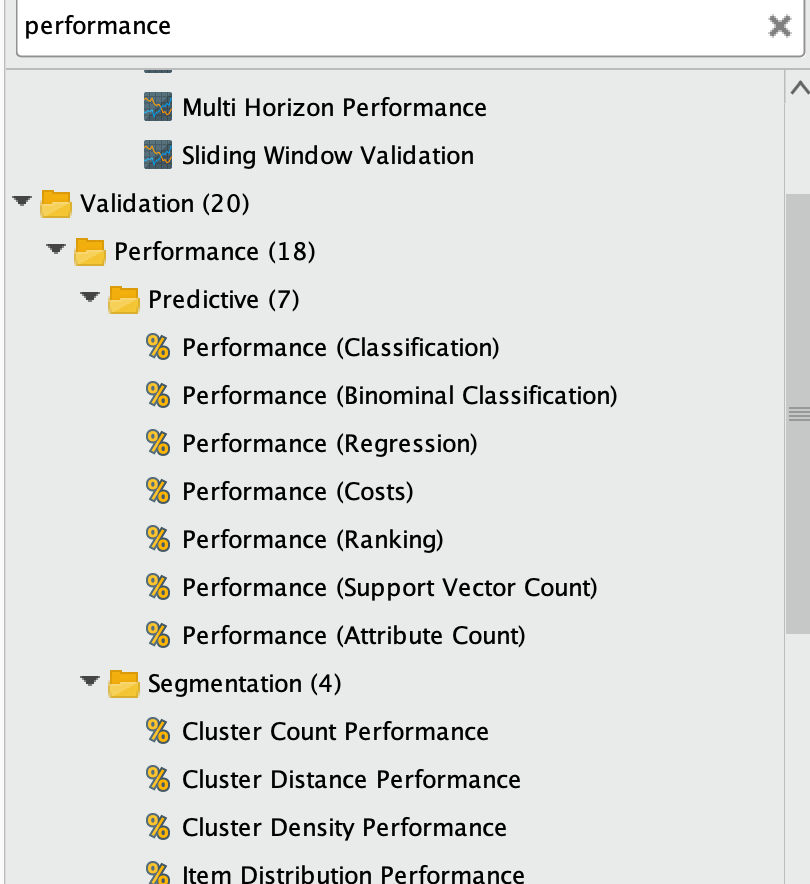In your example, RMSE is a commonly used error metric to measure the performance of regression models. I am not sure about the definitions of Margin or Soft Margin in the "Performance (Classification)". I will double check with the internal team and update later.
As a good reference, the log loss is defined here and commonly used in classification with the extra consideration of confidence values.
-log P(yt|yp) = -(yt log(yp) + (1 - yt) log(1 - yp))
https://www.quora.com/What-is-an-intuitive-explanation-for-the-log-loss-function

Cheers,
YY
• Member Posts: 11Contributor II
edited February 2020
I did that. My question concerns how technically one calculates the performance measures.
I have a decision tree which simply classifies instances. I am using gain ratio so I do not think it is a regression tree.
How do I calculate predicted value and then how do I calculate the difference between the predicted value and actual value.
Then, how do I calculate the margin and soft margin.
In a decision tree I see no probabilities associated with an individual instance. The tree simply classifies each instance to some class. So what is the predicted value. What is the margin - some minimum value of confidence from all the nodes in a tree?
I am using gain ratio to create the tree, but it is only to set the criteria in the nodes (or am I wrong? and I use it somehow to determine the margin or predicted value?).

• Member Posts: 11Contributor II
This is the model I am using. Inside of cross validation is a decision tree. I split the data sample and use a separate sample for secondary validation of the trained decision tree on a completely unknown instances.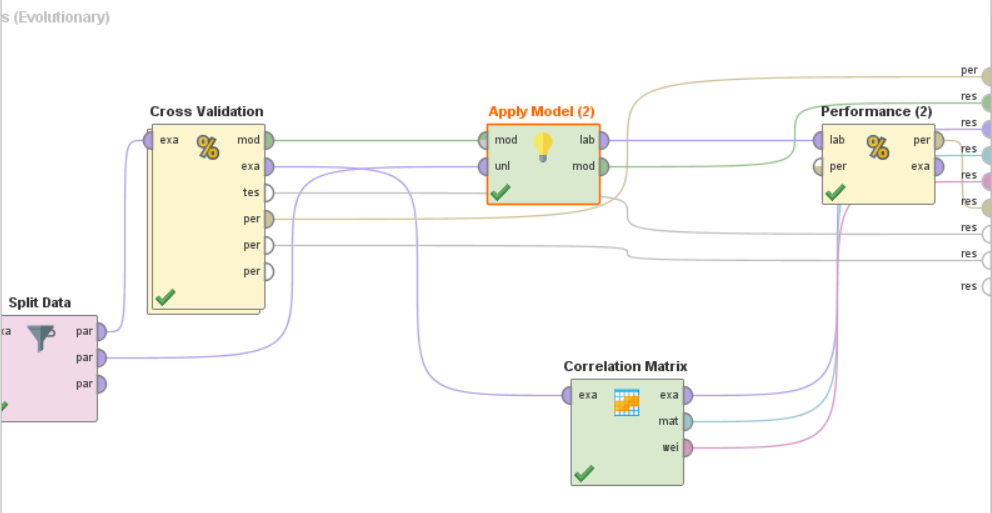• Member Posts: 11Contributor II
And here are the performance measures which are returned by the performance(2) element. I do not understand how they are calculated because I am using a binominal decision tree, not the regression tree and I have no idea how Performance(2) module calculates RMSE and other measures.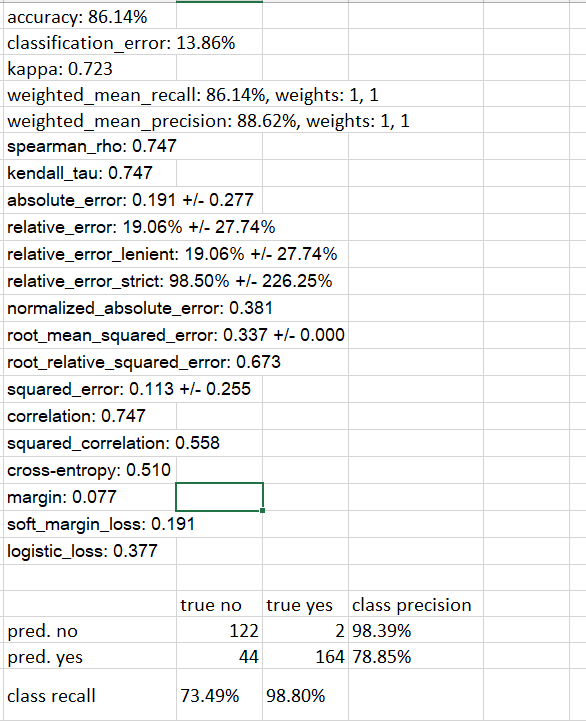• Member Posts: 11Contributor II
This is what I have inside of Cross-Validation module. I am training a binominal decision tree. Performance module which I have here returns only accuracy, precision and recall. This makes sense. I have no idea why the other module Performance(2) returns performance parameters which are suitable for regression trees, but not for binominal tree. I have no idea how it is possible that these measures are calculated.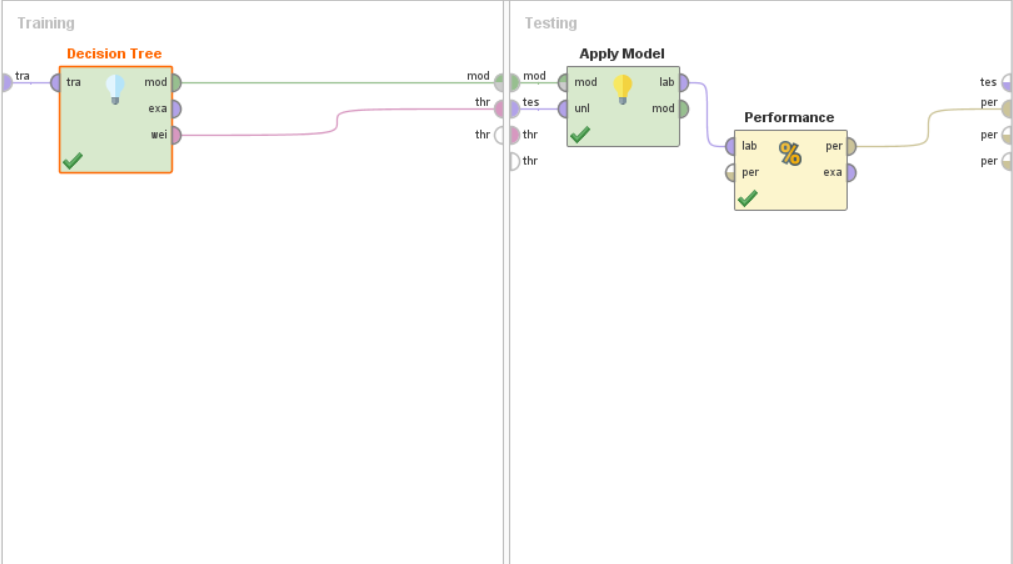• Member Posts: 11Contributor II
Here is what I see when I point a mouse on "per" entry in the Performance(2) module.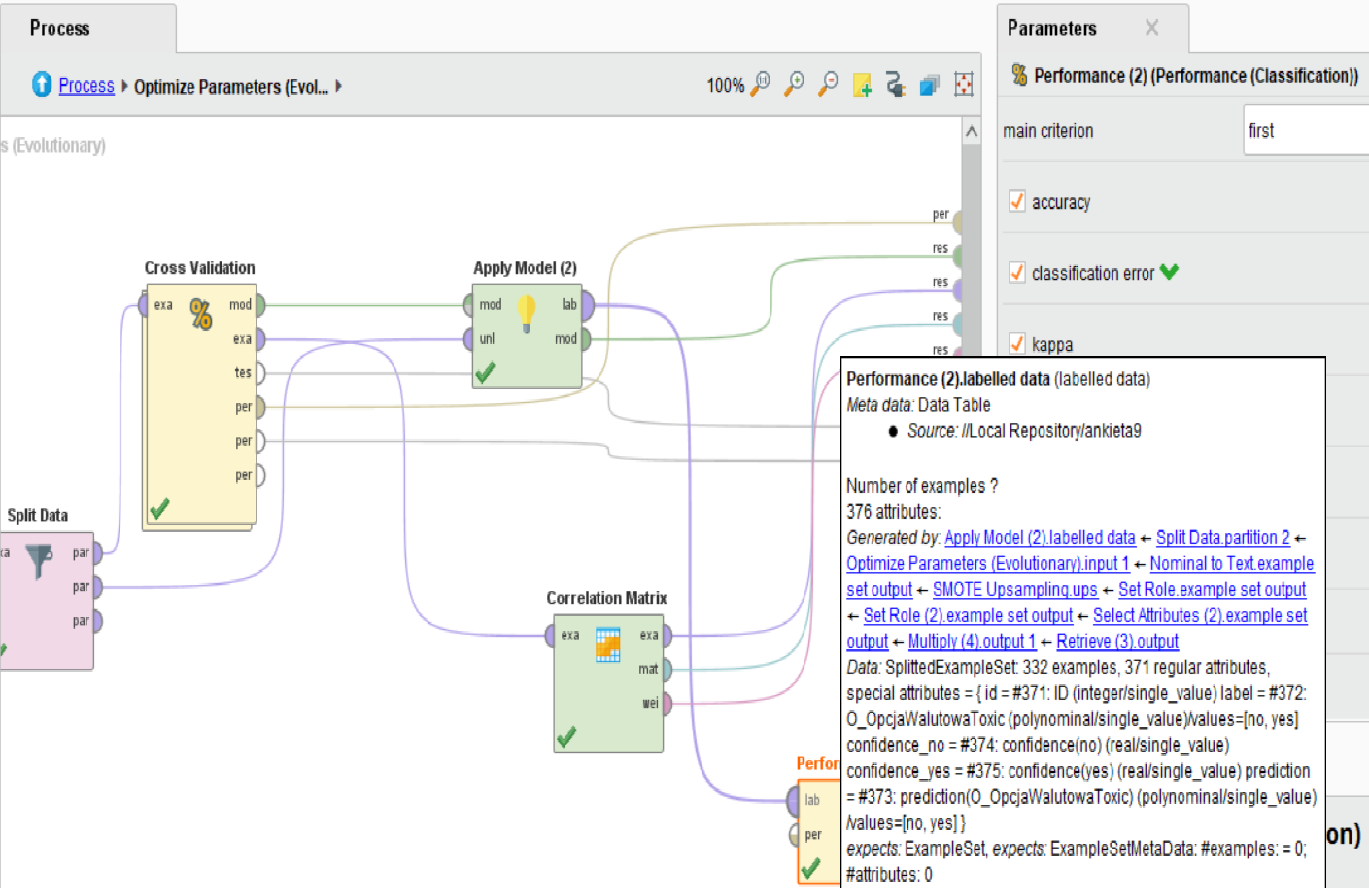• Member Posts: 11Contributor II
edited February 2020
So if I understand it right, these are the definitions for the performance parameters for the binominal tree?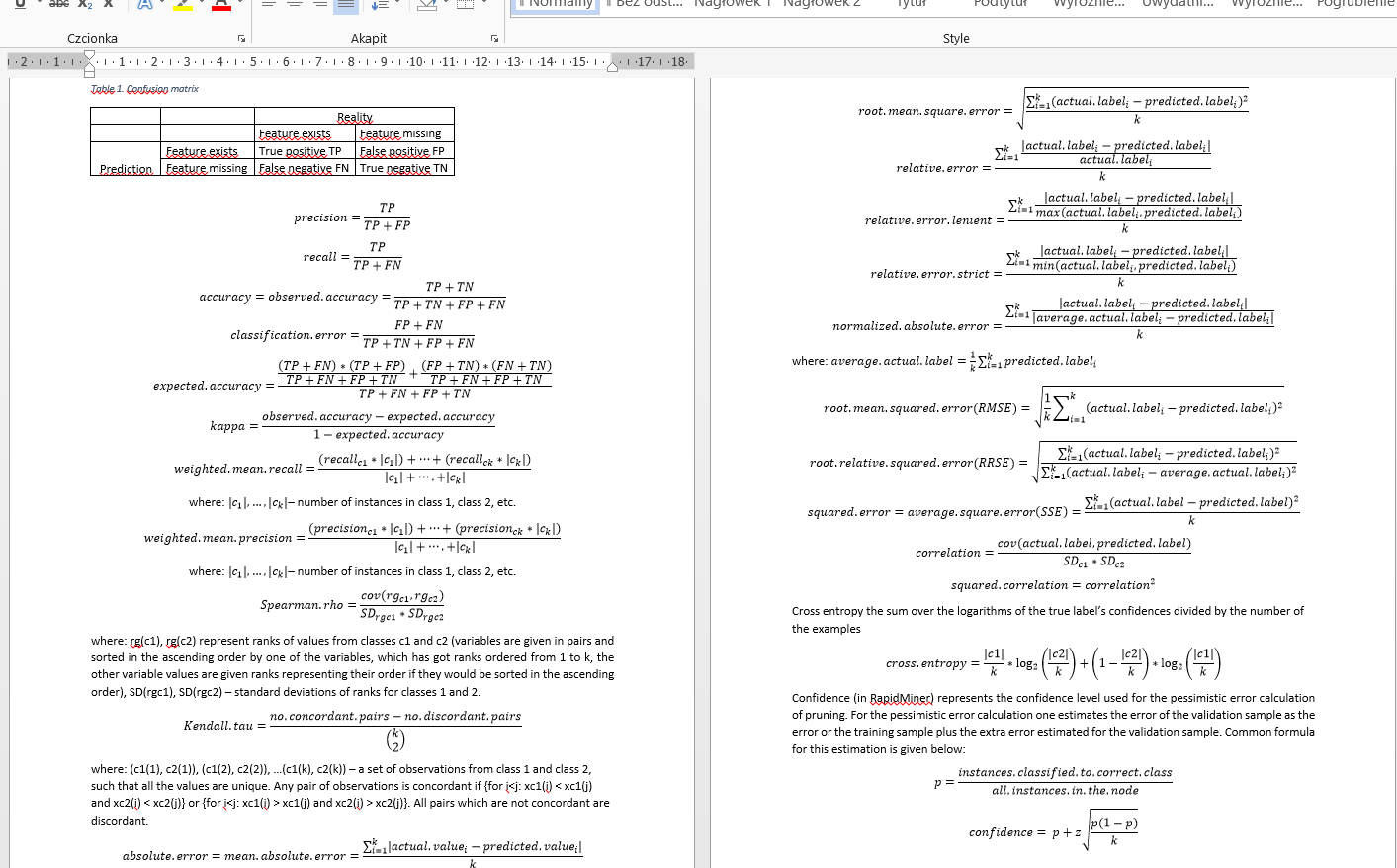• Member Posts: 11Contributor II
edited February 2020
I found the setter and getter for confidence in the example class.
However, if I understand it correctly, the Example class represents only 1 instance from the data set. So for every instance there is a separate value of confidence.
I do not know how it is calculated for every instance. In the decision tree I can set the confidence level (probably this is the z value from the normal distribution and it is used to calculate confidence for pruning). But if every instance has got its own confidence, then I do not know how it is calculated.